# Test Information Guide

## Field 51: Mathematics/Science (Middle School) Sample Multiple-Choice Questions

The following reference material will be available to you during the test:

Formulas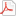Periodic Table### Number Sense and Operations

##### Objective 0002Understand principles and operations related to integers, fractions, decimals, percents, ratios, and proportions.

1. Use the sequence of steps below to answer the question that follows.

The sequence of steps above could be used to answer which of the following questions?

1. How can a decimal be converted to a fraction reduced to lowest terms?
2. Where should the decimal point be placed in the product of two decimals?
3. Why is it necessary to invert and multiply when solving problems involving fractions?
4. How is scientific notation used to multiply decimals?
Correct Response: B.

Correct Response: B.

### Patterns, Relations, and Algebra

##### Objective 0007Understand properties of functions and relations.

2. Use the diagram below to answer the question that follows.

Which of the following graphs represents the function described in the above diagram?

1.2.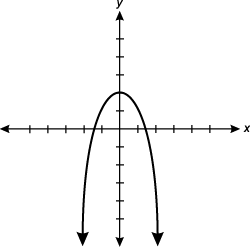3.4.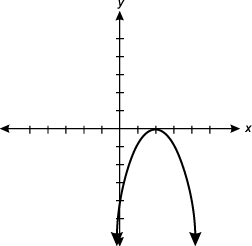Correct Response: B.

Correct Response: B.

### Geometry and Measurement

##### Objective 0012Understand the principles of Euclidean geometry and use them to prove theorems.

3. Use the geometric proof below to answer the question that follows.

Which of the following statements and reasons would be most appropriate in step 5 of this proof?

1.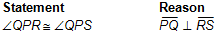2.3.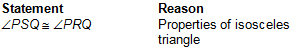4.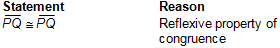Correct Response: D.

Correct Response: D.

### Data Analysis, Statistics, and Probability

##### Objective 0016Understand descriptive statistics and the methods used in collecting, organizing, reporting, and analyzing data.

4. Which of the following situations best represents a random sampling?

1. Ask every tenth person coming out of a health spa how many times a week they exercise to determine how often people in the town exercise.
2. Survey students in advanced biology classes to determine the average amount of time students in a certain school study each week.
3. Find the heights of all boys in a senior gym class to determine the average height of all boys in the school.
4. Count the number of chocolate chips in every fifth cookie to determine the average number of chocolate chips per cookie in a bag of cookies.
Correct Response: D.

Correct Response: D.

### Trigonometry, Calculus, and Discrete Mathematics

##### Objective 0019Understand the conceptual basis of calculus.

5. The graph of the function f(x) = 2x2 – 6x + 4 has its minimum value at the point (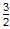, –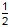). Which of the following statements must be true?

1. The derivative of f(x) is zero when x is equal to.
2. The value of f(x) approaches a limit of zero as x approaches.
3. The graph of f(x) shifts from a concave downward shape to a concave upward shape.
4. The slope of the line tangent to f(x) at x =is equal to –.
Correct Response: A.

Correct Response: A.

### History, Philosophy, and Methodology of Science

##### Objective 0022Understand principles and procedures of research and experimental design.

6. Students are planning to study the effects of a nitrogen fertilizer on the growth of young corn plants. Two weeks earlier, the students started approximately 100 plants from seed under identical conditions. From this group of 100 seedlings, the students will select 40 seedlings to use in their study. To provide experimental results that will be most representative of corn seedlings in general, the students should choose:

1. 20 large and 20 small seedlings.
2. 40 mid-sized seedlings.
3. 40 randomly selected seedlings.
4. the 40 smallest-looking seedlings.
Correct Response: C.

Correct Response: C.

### Chemistry

##### Objective 0028Understand the kinetic molecular model of matter.

7. Containers 1 and 2 are identical. Container 1 contains one mole of gas X, and container 2 contains one mole of gas Y. Both containers are sealed and represent closed systems. The molecules of the gases in the two containers have the same average speeds, but gas Y has a much higher temperature than gas X. Which of the following pairs of gases is possible for gas X and gas Y?

1. Gas X is helium, and gas Y is hydrogen.
2. Gas X is argon, and gas Y is hydrogen.
3. Gas X is oxygen, and gas Y is helium.
4. Gas X is oxygen, and gas Y is argon.
Correct Response: D.

Correct Response: D.

### Physics

##### Objective 0029Understand the concepts of force, motion, work, and power.

8. Use the diagram below to answer the question that follows.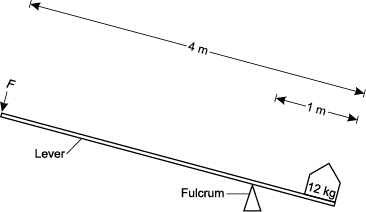The lever shown in the diagram above is 4 m long, with the fulcrum 1 m from one end. A 12 kg load is placed on the end of the lever nearest the fulcrum. What load must be applied to the opposite end of the lever at F in order to balance the 12 kg load?

1. 3 kg
2. 4 kg
3. 8 kg
4. 9 kg
Correct Response: B.

Correct Response: B.

### Biology

##### Objective 0034Understand principles related to the inheritance of characteristics.

9. A genetic mutation that results in a nucleotide insertion usually has a much greater effect on the organism than a genetic mutation that results in a nucleotide substitution. This is true because a nucleotide insertion:

1. causes a shift in the reading frame of the DNA code.
2. causes structural damage to the DNA molecule.
3. makes a gene unreadable at the ribosomal sites.
4. disrupts normal processes of mitosis and meiosis.
Correct Response: A.

Correct Response: A.

### Earth and Space Science

##### Objective 0037Understand geologic history and processes related to the changing earth.

10. Use the information below to answer the question that follows.

As Pangaea pulled apart, the modern Atlantic Ocean was formed. At the same time, another rift developed just to the west, along the present-day Connecticut River Valley. This rift, rather than forming an ocean or sea, resulted in volcanic activity and lava flows in the area that is known today as the Holyoke Range. Much of the old rift has since been filled in by sediment that later lithified.

Based on the information in this passage, the rock making up the Holyoke Range can best be classified as:

1. aggregate.
2. sedimentary rock.
3. metamorphic rock.
4. igneous rock.